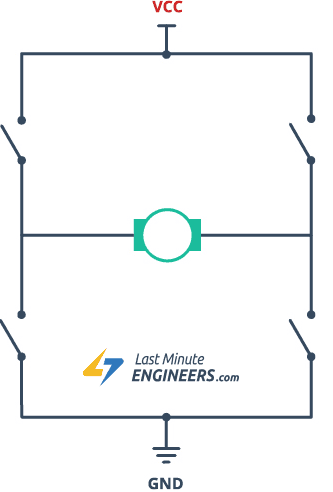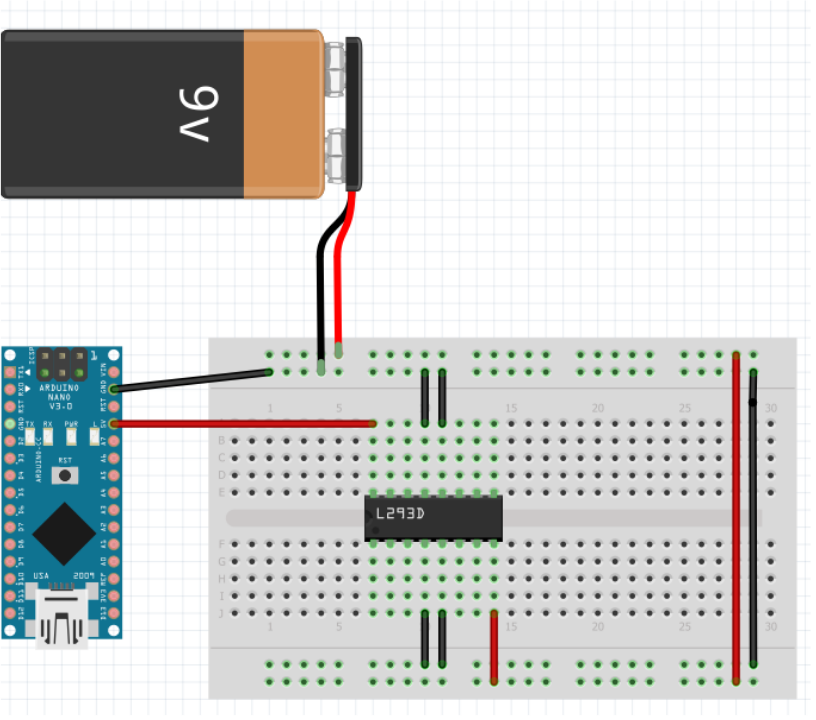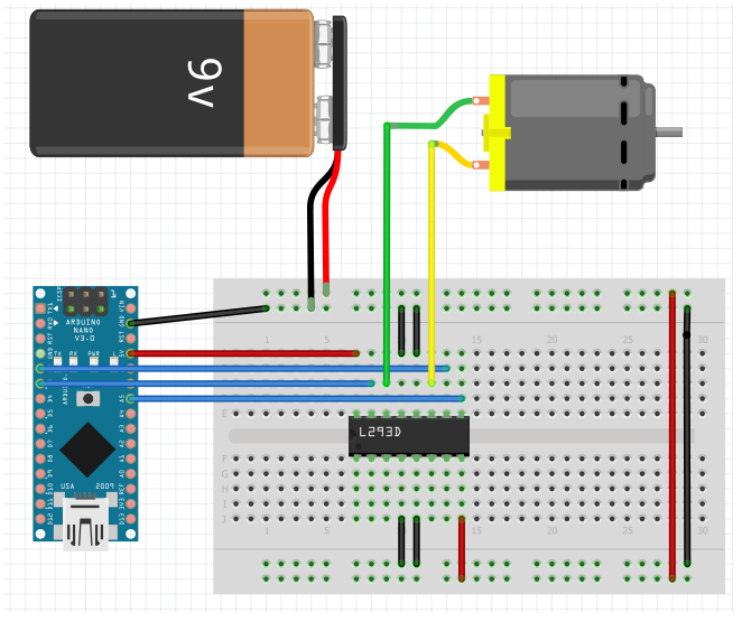top of page
• UFRJ Nautilus

# Digital Control of DC Motors

Updated: May 7, 2021

Drones, submarines or automobiles, all vehicles have one entity in common, without which it is impossible for it to work: the Motor. Indeed, a vehicle without mechanical materialization of electrical energy is unimaginable. In fact, there are several types of motors, but the focus of this article is the control and use of DC motors using digital signals.

The DC motor rotates in one direction when directly polarized and in the opposite direction when reversed polarized. You should imagine that like LEDs, buzzers and other simple electronic components, it is possible to connect them to the digital or analog ports on your microcontroller and control them by sending direct signals. Even if the voltage of the analog / digital ports is sufficient, the high current required by these components can (literally) fry the microcontroller. So, how to use motors, controlling their speed, direction of rotation and even using an external power supply?

To get around this situation we use a circuit known as the H-Bridge. An H-bridge is a circuit with the shape that resembles the letter H and is capable of altering the polarization of the central element through external signals. Turning it simple, this circuit has 4 switches that, when connected in certain combinations, allow current to flow in the motor in the desired direction, changing its direction of rotation. These switches can be replaced by electronic components, such as Transistors, which allows the polarity of the central element to depend exclusively on an external digital signal sent to the circuit.Circuito Ponte HPonte H usando Transistores

How to control motor using these concepts:

An usual implementation of the H bridge is done using IC l293D. This chip contains 2 H-bridges, through 4 ways, that is, it can control two independent motors, holding up to 600mAh in each and voltage from 4.5 to 36 Volts.L293 Pinout

The pins work as follows:

1,2EN -Activate channels 1 and 2

1A -4A: Input

1Y - 4Y -Output for motors

3,4EN -Activate channels 3 and 4

Ground -IC Ground

Vcc1 -IC Voltage Input

Vcc2 -Output Power for motors (From 4.5V to 36V)

1 - First we will power up IC using 5 volts of the Arduino (Vcc1), the power for the motors (Vcc2) and connect the grounds.Implementation of the H bridge - Part 1

2 - Now we connect 3,4EN and the Inputs 3A and 4A on the Arduino.Implementation of the H bridge - Part 2

3 - Now just connect the motor terminals to outputs 3Y and 4Y.Implementation of the H bridge - Part 3

### The code

We use the code below, which will be explained below, to implement the H bridge.

```int enable1 = A0;
int in1 = 2;
int in2 = 3;

//Executing only once
void setup(){
pinMode(enable1, OUTPUT);
pinMode(in1,OUTPUT);
pinMode(in2,OUTPUT);
}

//Executing in loop
void loop(){
//Define the motor speed
analogWrite(enable1, 255);
//Rotates the motor to one side for 1 second
digitalWrite(in1,HIGH);
digitalWrite(in2,LOW);
delay(1000);
//Rotates the motor to other side
digitalWrite(in1,LOW);
digitalWrite(in2,HIGH);
delay(1000);

}```

Detailing the Code:

```int enable1 = A0;
int in1 = 2;
int in2 = 3;```

We Declare the pins where we connected the inputs on IC.

```void setup(){
pinMode(enable1, OUTPUT);
pinMode(in1,OUTPUT);
pinMode(in2,OUTPUT);
}```

In function Setup we define the pins as OUTPUT.

`analogWrite(enable1, 255);`

The pins 3,4EN and 1,2EN, in addition to activating the respective channels can define the speed of the motors if a PWM signal is applied. 255 represents the maximum speed, that is, the total voltage applied to the L293D's Vcc2 pin.

```digitalWrite(in1,HIGH);
digitalWrite(in2,LOW);```

Now let's actually learn how to control the direction of rotation of the motors. The behavior of the motors is controlled by logic signals sent on the IC inputs. So, for our circuit:Assembly of the circuit with Arduino

Written by Gabriel Guimarães.